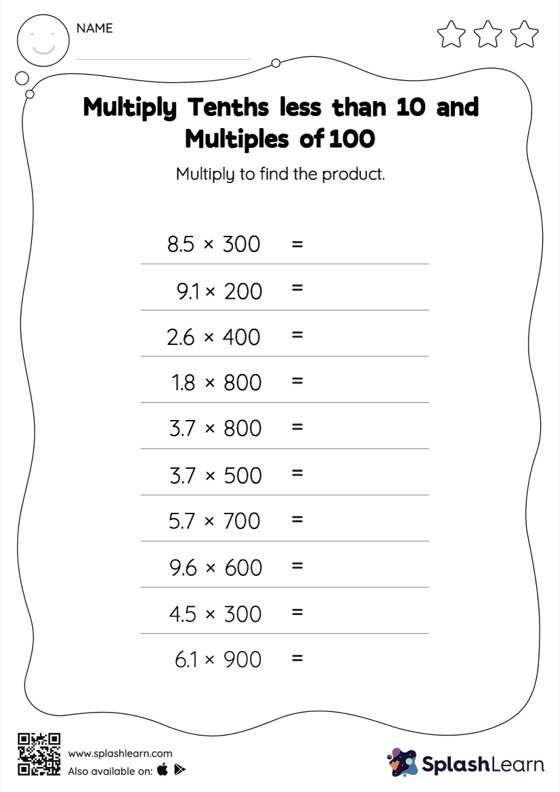# Multiply Tenths less than 10 and Multiples of 100: Horizontal Multiplication Worksheet

Home > Multiply Tenths less than 10 and Multiples of 100: Horizontal MultiplicationThis multiply tenths less than 10 and multiples of 100 worksheet consists of a set of questions on multiplication, which help students develop fluency. When students multiply a decimal by a number ending in zeros, they first ignore the decimal point, multiply the numbers, and then add the decimal point in the product as many places from the right as the number of decimal digits in the original number.